# Сборник текстов на казахском, русском, английском языках для формирования навыков по видам речевой деятельности обучающихся уровней среднего образования

 бет 28/65 Дата 05.11.2016 өлшемі 17,36 Mb.

## Ionic equations

An ionic equation is a chemical equation in which electrolytes are written as dissociated ions. Ionic equations are used for single and double displacement reactions that occur inaqueous solutions. For example, in the following precipitation reaction: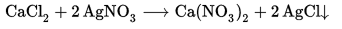{\displaystyle {\ce {{CaCl2}+2AgNO3->{Ca(NO3)2}+2AgCl(v)}}}

the full ionic equation is: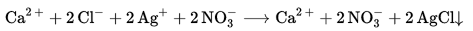{\displaystyle {\ce {{Ca^{2+}}+{2Cl^{-}}+{2Ag+}+2NO3^{-}->{Ca^{2+}}+{2NO3^{-}}+2AgCl(v)}}}

In this reaction, the Ca2+ and the NO3 ions remain in solution and are not part of the reaction. That is, these ions are identical on both the reactant and product side of the chemical equation. Because such ions do not participate in the reaction, they are called spectator ions. A net ionic equation is the full ionic equation from which the spectator ions have been removed. The net ionic equation of the proceeding reactions is:

{\displaystyle {\ce {{2Cl^{-}}+2Ag+->2AgCl(v)}}}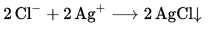or, in reduced balanced form,

{\displaystyle {\ce {{Ag+}+Cl^{-}->AgCl(v)}}}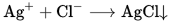In a neutralization or acid/base reaction, the net ionic equation will usually be:

H+(aq) + OH(aq) → H2O(l)

There are a few acid/base reactions that produce a precipitate in addition to the water molecule shown above. An example is the reaction of barium hydroxide with phosphoric acid, which produces not only water but also the insoluble salt barium phosphate. In this reaction, there are no spectator ions, so the net ionic equation is the same as the full ionic equation.

{\displaystyle {\ce {{3Ba(OH)2}+2H3PO4->{6H2O}+Ba3(PO4)2(v)}}}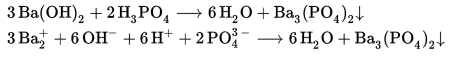{\displaystyle {\ce {{3Ba2+}+{6OH^{-}}+{6H+}+2PO4^{3-}->{6H2O}+Ba3(PO4)2(v)}}}Double displacement reactions that feature a carbonate reacting with an acid have the net ionic equation:

{\displaystyle {\ce {{2H+}+CO3^{2-}->{H2O}+CO2\uparrow }}}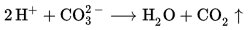If every ion is a "spectator ion" then there was no reaction, and the net ionic equation is null. 

An acid-base reaction is a chemical reaction that occurs between an acid and a base. Several concepts exist which provide alternative definitions for the reaction mechanisms involved and their application in solving related problems. Despite several similarities in definitions, their importance becomes apparent as different methods of analysis when applied to acid-base reactions for gaseous or liquid species, or when acid or base character may be somewhat less apparent. Historically, the first of these scientific concepts of acids and bases was provided by the French chemistAntoine Lavoisier, circa 1776.

Достарыңызбен бөлісу: